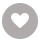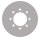# Learning Predictive Analytics with R电子书

37人正在读 | 0人评论9.8

• 读书简介
• 目录
• 累计评论(0条)
• 读书简介
• 目录
• 累计评论(0条)

Learning Predictive Analytics with R

Learning Predictive Analytics with R

Credits

www.PacktPub.com

Support files, eBooks, discount offers, and more

Why subscribe?

Free access for Packt account holders

Preface

Prediction

Supervised and unsupervised learning

Unsupervised learning

Supervised learning

Classification and regression problems

Classification

Regression

The role of field knowledge in data modeling

Caveats

What this book covers

What you need for this book

Who this book is for

Conventions

Customer support

Errata

Piracy

eBooks, discount offers, and more

Questions

1. Setting GNU R for Predictive Analytics

Installing GNU R

The R graphic user interface

The menu bar of the R console

A quick look at the File menu

A quick look at the Misc menu

Packages

Installing packages in R

Summary

2. Visualizing and Manipulating Data Using R

The roulette case

Histograms and bar plots

Scatterplots

Boxplots

Line plots

Application – Outlier detection

Formatting plots

Summary

3. Data Visualization with Lattice

Discovering multipanel conditioning with xyplot()

Discovering other lattice plots

Histograms

Stacked bars

Dotplots

Displaying data points as text

Updating graphics

Case study – exploring cancer-related deaths in the US

Discovering the dataset

Integrating supplementary external data

Summary

4. Cluster Analysis

Distance measures

Learning by doing – partition clustering with kmeans()

Setting the centroids

Computing distances to centroids

Computing the closest cluster for each case

Tasks performed by the main function

Internal validation

Using k-means with public datasets

Understanding the data with the all.us.city.crime.1970 dataset

Finding the best number of clusters in the life.expectancy.1971 dataset

External validation

Summary

5. Agglomerative Clustering Using hclust()

The inner working of agglomerative clustering

Agglomerative clustering with hclust()

Exploring the results of votes in Switzerland

The use of hierarchical clustering on binary attributes

Summary

6. Dimensionality Reduction with Principal Component Analysis

The inner working of Principal Component Analysis

Learning PCA in R

Dealing with missing values

Selecting how many components are relevant

PCA scores

Accessing the PCA scores

PCA scores for analysis

PCA diagnostics

Summary

7. Exploring Association Rules with Apriori

Apriori – basic concepts

Association rules

Itemsets

Support

Confidence

Lift

The inner working of apriori

Generating itemsets with support-based pruning

Generating rules by using confidence-based pruning

Analyzing data with apriori in R

Using apriori for basic analysis

Detailed analysis with apriori

Preparing the data

Analyzing the data

Coercing association rules to a data frame

Visualizing association rules

Summary

8. Probability Distributions, Covariance, and Correlation

Probability distributions

Introducing probability distributions

Discrete uniform distribution

The normal distribution

The Student's t-distribution

The binomial distribution

The importance of distributions

Covariance and correlation

Covariance

Correlation

Pearson's correlation

Spearman's correlation

Summary

9. Linear Regression

Understanding simple regression

Computing the intercept and slope coefficient

Obtaining the residuals

Computing the significance of the coefficient

Working with multiple regression

Analyzing data in R: correlation and regression

First steps in the data analysis

Performing the regression

Checking for the normality of residuals

Checking for variance inflation

Examining potential mediations and comparing models

Predicting new data

Robust regression

Bootstrapping

Summary

10. Classification with k-Nearest Neighbors and Naïve Bayes

Understanding k-NN

Working with k-NN in R

How to select k

Understanding Naïve Bayes

Working with Naïve Bayes in R

Computing the performance of classification

Summary

11. Classification Trees

Understanding decision trees

ID3

Entropy

Information gain

C4.5

The gain ratio

Post-pruning

C5.0

Classification and regression trees and random forest

CART

Random forest

Bagging

Conditional inference trees and forests

Installing the packages containing the required functions

Installing C4.5

Installing C5.0

Installing CART

Installing random forest

Installing conditional inference trees

Performing the analyses in R

Classification with C4.5

The unpruned tree

The pruned tree

C50

CART

Pruning

Random forests in R

Examining the predictions on the testing set

Conditional inference trees in R

Caret – a unified framework for classification

Summary

12. Multilevel Analyses

Nested data

Multilevel regression

Random intercepts and fixed slopes

Random intercepts and random slopes

Multilevel modeling in R

The null model

Random intercepts and fixed slopes

Random intercepts and random slopes

Predictions using multilevel models

Using the predict() function

Assessing prediction quality

Summary

13. Text Analytics with R

An introduction to text analytics

Data preparation

Preprocessing and inspecting the corpus

Computing new attributes

Creating the training and testing data frames

Classification of the reviews

Document classification with k-NN

Document classification with Naïve Bayes

Classification using logistic regression

Document classification with support vector machines

Mining the news with R

A successful document classification

Extracting the topics of the articles

Collecting news articles in R from the New York Times article search API

Summary

14. Cross-validation and Bootstrapping Using Caret and Exporting Predictive Models Using PMML

Cross-validation and bootstrapping of predictive models using the caret package

Cross-validation

Performing cross-validation in R with caret

Bootstrapping

Performing bootstrapping in R with caret

Predicting new data

Exporting models using PMML

What is PMML?

A brief description of the structure of PMML objects

Examples of predictive model exportation

Exporting k-means objects

Hierarchical clustering

Exporting association rules (apriori objects)

Exporting Naïve Bayes objects

Exporting decision trees (rpart objects)

Exporting random forest objects

Exporting logistic regression objects

Exporting support vector machine objects

Summary

A. Exercises and Solutions

Exercises

Chapter 1 – Setting GNU R for Predictive Modeling

Chapter 2 – Visualizing and Manipulating Data Using R

Chapter 3 – Data Visualization with Lattice

Chapter 4 – Cluster Analysis

Chapter 5 – Agglomerative Clustering Using hclust()

Chapter 6 – Dimensionality Reduction with Principal Component Analysis

Chapter 7 – Exploring Association Rules with Apriori

Chapter 8 – Probability Distributions, Covariance, and Correlation

Chapter 9 – Linear Regression

Chapter 10 – Classification with k-Nearest Neighbors and Naïve Bayes

Chapter 11 – Classification Trees

Chapter 12 – Multilevel Analyses

Chapter 13 – Text Analytics with R

Solutions

Chapter 1 – Setting GNU R for Predictive Modeling

Chapter 2 – Visualizing and Manipulating Data Using R

Chapter 3 – Data Visualization with Lattice

Chapter 4 – Cluster Analysis

Chapter 5 – Agglomerative Clustering Using hclust()

Chapter 6 – Dimensionality Reduction with Principal Component Analysis

Chapter 7 – Exploring Association Rules with Apriori

Chapter 8 – Probability Distributions, Covariance, and Correlation

Chapter 9 – Linear Regression

Chapter 10 – Classification with k-Nearest Neighbors and Naïve Bayes

Chapter 11 – Classification Trees

Chapter 12 – Multilevel Analyses

Chapter 13 – Text Analytics with R

Preface

Chapter 1 – Setting GNU R for Predictive Modeling

Chapter 2 – Visualizing and Manipulating Data Using R

Chapter 3 – Data Visualization with Lattice

Chapter 4 – Cluster Analysis

Chapter 5 – Agglomerative Clustering Using hclust()

Chapter 6 – Dimensionality Reduction with Principal Component Analysis

Chapter 7 – Exploring Association Rules with Apriori

Chapter 8 – Probability Distributions, Covariance, and Correlation

Chapter 9 – Linear Regression

Chapter 10 – Classification with k-Nearest Neighbors and Naïve Bayes

Chapter 11 – Classification Trees

Chapter 12 – Multilevel Analyses

Chapter 13 – Text Analytics with R

Chapter 14 – Cross-validation and Bootstrapping Using Caret and Exporting Predictive Models Using PMML

Index

01

Simon Holmes
￥122.99
02

Chris Khoo
￥70.99
03

Debarchan Sarkar
￥122.99
04

Tyler L. Longren
￥100.99
05

Simone Scarduzio
￥73.99
06

Maxwell Shinn
￥61.99
07

### PostgreSQL Replication￥152.99

Zoltan B?sz?rmenyi
￥152.99
08

Michael Peacock
￥100.99
09

Ahmed Lashin
￥167.99
10

### Mastering Apple Aperture￥152.99

Thomas Fitzgerald
￥152.99
•关注我们

最受欢迎的阅读产品

关注我们：

•关于我们

欢迎反馈宝贵意见给我们

客服书吧：当当云阅读问答